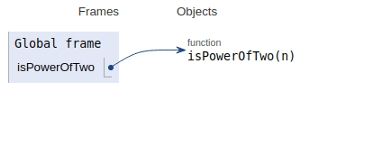# Python Program to find whether a no is the power of two

In this article, we will learn about the solution and approach to solve the given problem statement.

## Problem statement

Given a number n, we need to check whether the given number is a power of two.

## Approach

• Continue dividing the input number by two, i.e, = n/2 iteratively.

• We will check In each iteration, if n%2 becomes non-zero and n is not 1 then n is not a power of 2.

• If n becomes 1 then it is a power of 2.

Let’s see the implementation below −

## Example

def isPowerOfTwo(n):
if (n == 0):
return False
while (n != 1):
if (n % 2 != 0):
return False
n = n // 2
return True
# main
if(isPowerOfTwo(40)):
print('Yes')
else:
print('No')

## Output

No

All the variables and functions are declared in the global scope as shown below −## Conclusion

In this article, we learned about the approach to find whether a number is power of two or not.